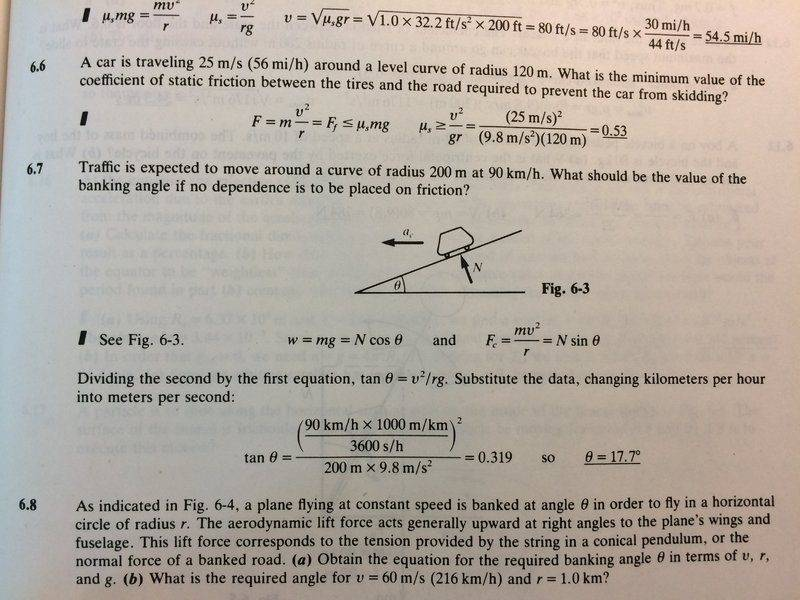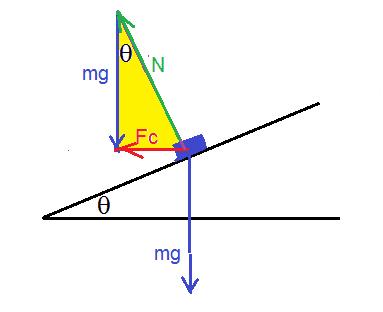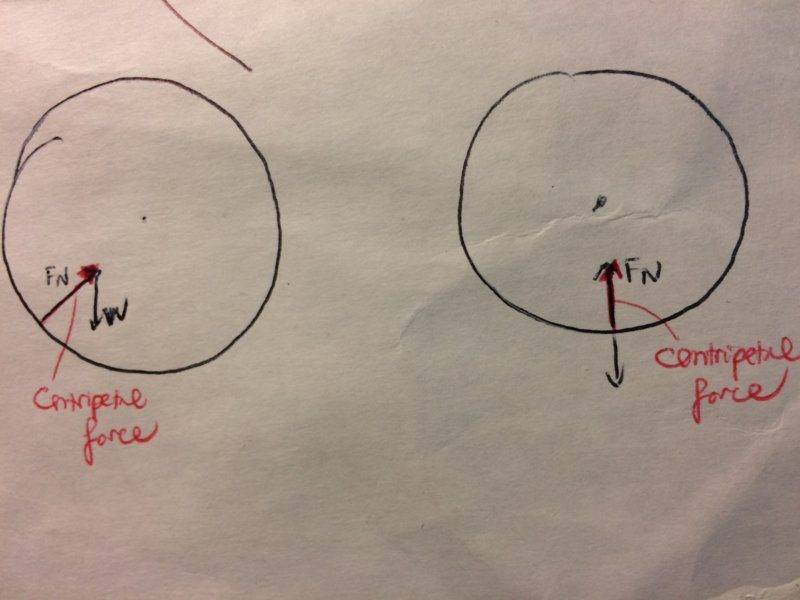# Centripetal Force: Find banking angle on a roadway

## Homework Statement

Problem 6.7[/B]

Speed: 90 km/h=90000m/h

What should be the value of the banking angle if no dependence is to be placed on friction?## Homework Equations

##F=ma##

##F_c=\frac {mv^2} {r}##

##w=mg##

Trig functions

## The Attempt at a Solution

I got an answer that is flipped from the book’s key. My work is below:

N=normal force

##N=F_c * sin \theta = \frac {mv^2} {r}* sin \theta ##

##N=w*cos \theta=mg*cos\theta##

##\frac {mv^2} {r}* sin \theta = mg*cos\theta##

##\frac {sin \theta} {cos\theta} =tan \theta= \frac {gr} {v^2}##

However, the key says that ##tan\theta= \frac {v^2} {gr}## For example I’m particularly not sure how they set up the equation ##w= N cos \theta## .I thought it should be ## N= w cos\theta##? I tried to draw out the triangle diagram but still can't figure it out.

Thank you!

#### Attachments

FactChecker
Gold Member
Try a quick "sanity check" of your equation ## N = w\cos\theta##. When the car is going around a banked turn at a huge speed, ##N## can be very large -- much larger than ##w##. But your equation forces ##N \le w## since ##\cos\theta \le 1##. So you should review your logic that led to that equation.

Consider two cases:
1) When ##\theta = 0##, there is no bank and ##N = w##.
2) As ##\theta## increases to 90, ##N## is always greater than ##w## and goes to infinity.

•Beth N
haruspex
Homework Helper
Gold Member
##N=w*cos \theta=mg*cos\theta##
Further to @FactChecker's reply, to get that equation you would have considered force and acceleration components normal to the slope. But it is not complete.
In an inertial frame, it omits a component of the resulting acceleration.
In the frame of the car, it omits a component of centrifugal force.

•FactChecker
ehild
Homework Helper

## The Attempt at a Solution

I got an answer that is flipped from the book’s key. My work is below:

N=normal force

##N=F_c * sin \theta = \frac {mv^2} {r}* sin \theta ##

##N=w*cos \theta=mg*cos\theta##

##\frac {mv^2} {r}* sin \theta = mg*cos\theta##

##\frac {sin \theta} {cos\theta} =tan \theta= \frac {gr} {v^2}##
Always make a drawing!
Do not forget that the net force on the car must be equal to the centripetal force. It is the sum of the normal force and the weight. See the yellow triangle. So your formulas are wrong.#### Attachments

•FactChecker and Beth N
Oh I see! From looking at ehild's diagram I realized that I made the mistake of calling normal force a cosine component of ##F_c## instead of weight. Still, I completely misunderstood that the normal force should be smaller than the weight, like in a static object resting on an incline.
2) As θθ\theta increases to 90, NNN is always greater than www and goes to infinity
I'll need to review facts about circular motion. Would this situation be analogous to a car on a roller coaster approaching the bottom? (where normal force is greater than weight)

In the frame of the car, it omits a component of centrifugal force.
I'm not too familiar with what you're saying but I saw in this video it says that centrifugal force does not exist? (@ 7:49)

haruspex
Homework Helper
Gold Member
I saw in this video it says that centrifugal force does not exist? (@ 7:49)
That's overstating it. It's to do with frames of reference.

In an inertial frame (one that is neither accelerating nor rotating) there is no centrifugal force. The net force (of the normal force and gravitation) results in the acceleration observed in the inertial frame, namely, centripetal acceleration.

In the car's frame of reference there is, by definition, no acceleration. Yet the known forces do not balance. We can fix this up by inventing a pseudo force we call centrifugal force. It's just a different way of looking at things and both produce correct answers to physics questions.
In the same way, passengers in a car accelerating in a straight line feel thrown back against their seats. In their (accelerating) frame that is a pseudo force balancing the normal forces from the seat backs.

•Beth N
ehild
Homework Helper
Oh I see! From looking at ehild's diagram I realized that I made the mistake of calling normal force a cosine component of ##F_c## instead of weight. Still, I completely misunderstood that the normal force should be smaller than the weight, like in a static object resting on an incline.
If you look at the diagram, the weight is the cosine component of the normal force and Fc is the sine component of it.
You have to know, that the normal force is perpendicular to the road, the weight points vertically downward and the centripetal force is horizontal, pointing inward. All forces are sides of a right triangle, and the normal force is the hypotenuse, so the biggest of the sides.
This is not a static object, resting on an incline, but one moving along a horizontal circle on an inclined road. The motion can only happen if the appropriate centripetal force acts on the car; and that force is provided by the horizontal component of the normal force. The vertical component opposes the weight.

•Beth N
PeterO
Homework Helper
In the frame of the car, it omits a component of centrifugal force.

I don't think you should consider the problem from the frame of the car. The car is acccelerating (travelling in a circle) and thus in a non inertial frame - that is the only reason the ficticious force (centrifugal) raises its non-existant head.

•Beth N
FactChecker
Gold Member
I'll need to review facts about circular motion. Would this situation be analogous to a car on a roller coaster approaching the bottom? (where normal force is greater than weight)
I wouldn't say it's like that exactly. Suppose the bank angle, ##\theta##, increases toward 90 with no lateral force as the speed around the curve increases. That means that the centripetal force is growing so much that the gravitational force is a smaller and smaller part of the total normal force. For that to happen, the speed is increasing so much that the centripetal force is becoming larger and larger. It can get as large as you want. The normal force is that plus the gravitational force, so it is always greater than either the centripetal or gravitational force alone.

Last edited:
•Beth N
I wouldn't say it's like that exactly. Suppose the bank angle, θθ\theta, increases toward 90 with no lateral force as the speed around the curve increases. That means that the centrifugal force is growing so much that the gravitational force is a smaller and smaller part of the total normal force. For that to happen, the speed is increasing so much that the centrifugal force is becoming larger and larger. It can get as large as you want. The normal force is that plus the gravitational force, so it is always greater than either the centrifugal or gravitational force alone.

Thanks for the explanation! So for the roller coaster, the normal force is independent of the gravitational force and the centripetal force? (They're not forming a triangle as in the banked road..)

FactChecker
Gold Member
Thanks for the explanation! So for the roller coaster, the normal force is independent of the gravitational force and the centripetal force? (They're not forming a triangle as in the banked road..)
The normal force is the sum of the gravitational force (down) and the centripetal force (horizontal). The 3 forces do form a right triangle.

Note: I said centrifugal several times above when I should have said centripetal. They are equal and opposite. I will correct my post above.

Last edited:
haruspex
Homework Helper
Gold Member
I don't think you should consider the problem from the frame of the car. The car is acccelerating (travelling in a circle) and thus in a non inertial frame - that is the only reason the ficticious force (centrifugal) raises its non-existant head.
It is a perfectly valid method. I rarely use it myself, but I have seen examples where it produces the most elegant solution.

The normal force is the sum of the gravitational force (down) and the centripetal force (horizontal). The 3 forces do form a right triangle.

I still don't get why? Wouldn't the centripetal force points in the same direction as the normal force in a roller coaster (where the circular path of travel is vertical to the ground)?#### Attachments

FactChecker
Gold Member
Sorry, you are right. I was still talking about the situation of a car traveling in a horizontal loop in a constant turn. Then the gravitational force is down and the centripetal force is horizontal at a right angle. The normal force is (in this problem) the sum of the two forces and is the hypotenuse of the right triangle (because the bank angle of the turn is calculated in this problem to make the normal force line up with the total force.)

In the case of the roller coaster, your diagrams look good to me. There is no right angle between the centripetal force and the gravitational force (except when the car is exactly at the height of the center). And when the car is at the bottom, the two forces are aligned but in opposite directions, as your diagram shows.

Last edited:
•Beth N
Sorry, you are right. I was still talking about the situation of a car traveling in a horizontal loop in a constant turn. Then the gravitational force is down and the centripetal force is horizontal at a right angle. The normal force is the sum of the two forces and is the hypotenuse of the right triangle.

In the case of the roller coaster, your diagrams look good to me. There is no right angle between the centripetal force and the gravitational force (except when the car is exactly at the height of the center). And when the car is at the bottom, the two forces are aligned but in opposite directions, as your diagram shows.
I see, thank you!

FactChecker